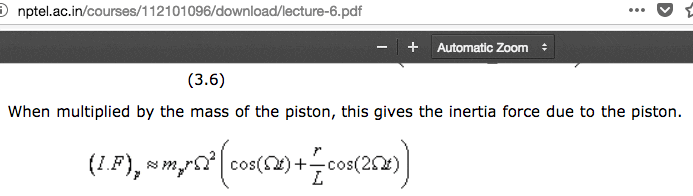# Help identifying symbol

• I
• Jaguar7555

#### Jaguar7555

Here is the formula in the screenshot figuring piston assembly inertia.
piston assembly inertia = Reciprocating Mass * CRANK RADIUS * W^2*(COS(Wt)+(crank radius/rod length)*COS(2*Wt))
W=angular frequency (crank rotations in 1 second times 6.28)
Wt=unknown

Please identify the Wt (omega t) symbol. thanks

Here's the screenshot: